# LeetCode 75 Study Plan

This study plan is for those who want to prepare for technical interviews but are uncertain which problems they should focus on. The problems have been carefully curated so that levels 1 and 2 will guide beginner and intermediate users through problems that cover the data structures and algorithms necessary to succeed in interviews with most mid-tier companies. While level 3 provides material to help users whose targets are top-tier companies. -- LeetCode

## Day 1 Prefix Sum​

### 724. Find Pivot Index​

note

At a given index i, how do I get the value of leftSum and rightSum?

Ans:

• leftSum of i-th element can be computed by summing up all the values on the left of the i-th element.
• rightSum of i-th element is equal to the sum of the array minus the value of the current element and leftSum.

## Day 2 String​

### 205. Isomorphic Strings​

note

Given two strings s and t, determine if they are isomorphic.

Two strings s and t are isomorphic if the characters in s can be replaced to get t.

All occurrences of a character must be replaced with another character while preserving the order of characters. No two characters may map to the same character, but a character may map to itself.

Should pay more attention on the last paragraph of the requirement. A character can only be mapped to one character. Therefore, create a set to store the values. i.e. one-to-one relationship.

inputmapresult
egg -> add{e : a, g : d}true
1. b -> b
2. a -> a
3. d -> b
4. c -> a
false

For "badc -> baba", it has two characters that map to b (b and d) so it is not isomorphic.

### 392. Is Subsequence​

note

Given two strings s and t, return true if s is a subsequence of t, or false otherwise.

A subsequence of a string is a new string that is formed from the original string by deleting some (can be none) of the characters without disturbing the relative positions of the remaining characters. (i.e., "ace" is a subsequence of "abcde" while "aec" is not).

A simple example isSubsequence("bc", "abagdc")

First, check if b in the string if it does exist then check the remaining characters to see if c exist.

### 21. Merge Two Sorted Lists​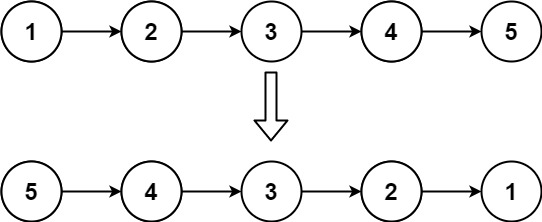tip

Change each node's next pointer to its left node.

null <- 1 <- 2 <- 3

### 876. Middle of the Linked List​

note

If there are two middle nodes, return the second middle node.

Example 1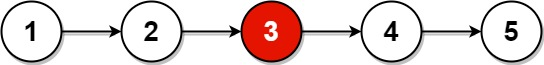Input: head = [1,2,3,4,5]Output: [3,4,5]Explanation: The middle node of the list is node 3.

Example 2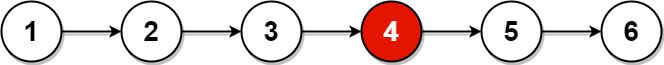Input: head = [1,2,3,4,5,6]Output: [4,5,6]Explanation: Since the list has two middle nodes with values 3 and 4, we return the second one.
tip

Fast and Slow Pointer

When traversing the list with a pointer slow, make another pointer fast that traverses twice as fast.

• slow = slow.next
• fast = fast.next.next

When fast reaches the end of the list, slow must be in the middle.

### 142. Linked List Cycle II​

note

Given the head of a linked list, return the node where the cycle begins. If there is no cycle, return null.

## Day 5 Greedy​

### 121. Best Time to Buy and Sell Stock​

Two import information to keep track of:

• min price
• max profit

### 409. Longest Palindrome​

note

Given a string s which consists of lowercase or uppercase letters, return the length of the longest palindrome that can be built with those letters.

Imagine we build a palindrome string, it will have as many as partnered letters as possible, plus possibly a unique center character.

• If the number of occurrences of a letter is even, add the count to the total length of the palindrome.
• If the number of occurrences of a letter is odd, subtract the count by 1 and add it to the total length of the palindrome.
• If the palindrome haven't added a unique character to the center, add 1 to the length of the palindrome if a character with the number of occurrences equals 1 is found.
• e.g. {b : 2, f : 1, c : 1}, f can be added to the center of the palindrome and it is the only character that is unique.
warning
• We can only insert 1 unique character and it has to be at the center.
• aa, abba are palindrome strings. The center of the palindrome can have 2 characters.

## Day 6 Tree​

### 589. N-ary Tree Preorder Traversal​

note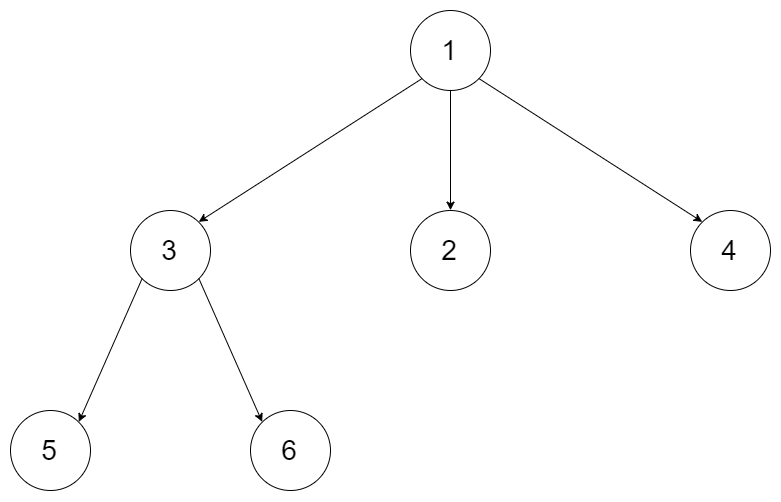• Input: root = [1,null,3,2,4,null,5,6]
• Output: [1,3,5,6,2,4]

Pre-order traversal is root -> left -> right.

### 102. Binary Tree Level Order Traversal​

note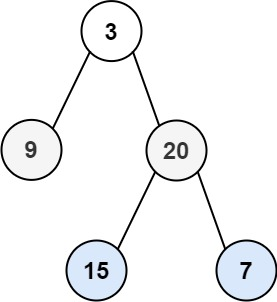• Input: root = [3,9,20,null,null,15,7]
• Output: [,[9,20],[15,7]]
• Enqueue all nodes of a level.
• Process the tree level-by-level.
• Enqueue all children of each node in the current level.
• How to process level-by-level? This is achieved by checking the number of nodes in a level before processing a level.
note

Given an array of integers nums which is sorted in ascending order, and an integer target, write a function to search target in nums. If target exists, then return its index. Otherwise, return -1.

Input: nums = [-1,0,3,5,9,12], target = 9Output: 4Explanation: 9 exists in nums and its index is 4
Input: nums = [-1,0,3,5,9,12], target = 2Output: -1Explanation: 2 does not exist in nums so return -1

## Day 8 Binary Search Tree​

### 98. Validate Binary Search Tree​

note

Given the root of a binary tree, determine if it is a valid binary search tree (BST).

A valid BST is defined as follows:

• The left subtree of a node contains only nodes with keys less than the node's key.
• The right subtree of a node contains only nodes with keys greater than the node's key.
• Both the left and right subtrees must also be binary search trees.

Example 1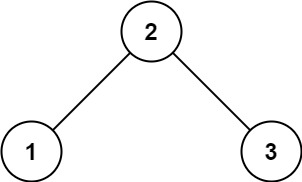Input: root = [2,1,3]Output: trueInput: root = [5,1,4,null,null,3,6]Output: falseExplanation: The root node's value is 5 but its right child's value is 4.

#### Solution 1​

solution

Main idea: Check if the current node's value is within the valid range (floor/ceiling).

• Base case: root=null, the tree is a valid binary search tree.
• Recursively check the valid range of each node floor < root.val < ceiling:
• Initially, the valid range of the root node is $[\infty, +\infty]$
• After visiting the root, we start restricting the valid range.
• If we move to the left child, the ceiling of the left child will be updated to root.val.
• If we move to the right child, the floor of the right child will be updated to root.val.
var isValidBST = function (root, floor = -Infinity, ceiling = Infinity) {  if (!root) return true;  if (!(floor < root.val && root.val < ceiling)) {    return false;  }  return (    isValidBST(root.left, floor, root.val) &&    isValidBST(root.right, root.val, ceiling)  );};

#### Solution 2​

Use in-order traversal to collect all the values of a binary search tree, then check if the values are in the correct order (ascending).

### 235. Lowest Common Ancestor of a Binary Search Tree​

note

Given a binary search tree (BST), find the lowest common ancestor (LCA) node of two given nodes in the BST.

“The lowest common ancestor is defined between two nodes p and q as the lowest node in Tree that has both p and q as descendants (where we allow a node to be a descendant of itself).”

Example 1: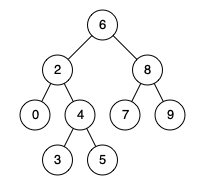Input: root = [6,2,8,0,4,7,9,null,null,3,5], p = 2, q = 8Output: 6Explanation: The LCA of nodes 2 and 8 is 6.

Example 2:Input: root = [6,2,8,0,4,7,9,null,null,3,5], p = 2, q = 4Output: 2Explanation: The LCA of nodes 2 and 4 is 2,since a node can be a descendant of itself according to the LCA definition.

#### Solution 1​

• Level order traversal, callback(node, levelNum)
• Search for the target nodes based on the current node.
• Found target nodes - update the lowest common ancestor

#### Solution 2​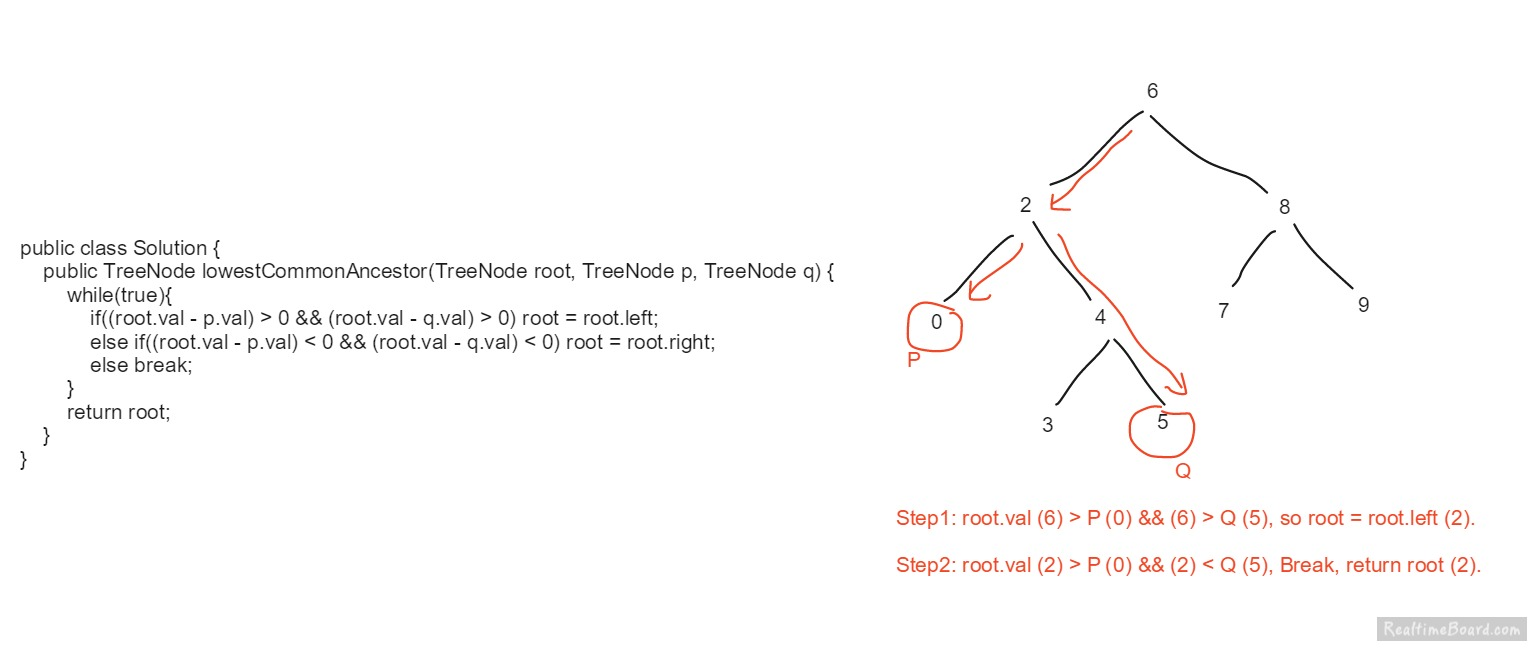• If both target nodes are less than the current node, pick the left child as the potential common ancestor.
• If both target nodes are greater than the current node, pick the right child as the potential common ancestor.
• If the current node is causing a diverging, return the current node. e.g. 0 and 5 go for different subtrees at node 2. So node 2 is the lowest common ancestor.
info

There are some special constraints:

• All Node.val are unique.
• p != q
• p and q will exist in the BST.

## Day 9 Graph/BFS/DFS​

### 733. Flood Fill​

• Array
• Depth-First Search
note

You are given three integers sr, sc, and color. You should perform a flood fill on the image starting from the pixel image[sr][sc].

• sr: starting row
• sc: starting col

To perform a flood fill, consider the starting pixel, plus any pixels connected 4-directionally to the starting pixel of the same color as the starting pixel, plus any pixels connected 4-directionally to those pixels (also with the same color), and so on. Replace the color of all of the aforementioned pixels with color.

Example 1: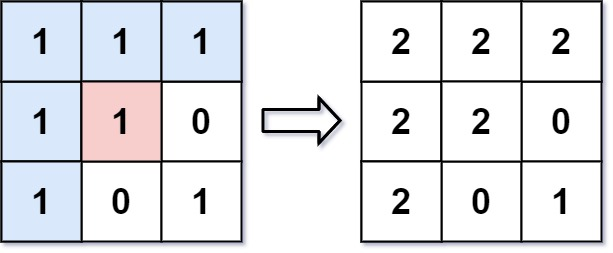Input: image = [[1,1,1],[1,1,0],[1,0,1]], sr = 1, sc = 1, color = 2Output: [[2,2,2],[2,2,0],[2,0,1]]

Example 2:

Input: image = [[0,0,0],[0,0,0]], sr = 0, sc = 0, color = 0Output: [[0,0,0],[0,0,0]]

### 200. Number of Islands​

• Array
• Depth-First Search
note

Given an m x n 2D binary grid grid which represents a map of '1's (land) and '0's (water), return the number of islands.

An island is surrounded by water and is formed by connecting adjacent lands horizontally or vertically. You may assume all four edges of the grid are all surrounded by water.

#### Solution​

https://leetcode.com/problems/number-of-islands/discuss/56340/Python-Simple-DFS-Solution

Iterate through each of the cell and if it is a land, do DFS to mark the all adjacent lands, then increment the counter by 1.

def numIslands(self, grid):    if not grid:        return 0    count = 0    for i in range(len(grid)):        for j in range(len(grid)):            if grid[i][j] == '1':                self.dfs(grid, i, j)                count += 1    return countdef dfs(self, grid, i, j):    if i<0 or j<0 or i>=len(grid) or j>=len(grid) or grid[i][j] != '1':        return    grid[i][j] = '#' # mark/exclude the entire land, only count it once in the main loop    self.dfs(grid, i+1, j)    self.dfs(grid, i-1, j)    self.dfs(grid, i, j+1)    self.dfs(grid, i, j-1)

## Day 10 Dynamic Programming​

### 70. Climbing Stairs​

def climbStairs(n):    if n == 1:        return 1    if n == 2:        return 2    return climbStairs(n-1) + climbStairs(n-2)

## Day 11 Dynamic Programming​

Dynamic Programming - Learn to Solve Algorithmic Problems

## Day 12 Sliding Window/Two Pointer​

### 438. Find All Anagrams in a String​

Use sliding window technique, which is inspired by this https://leetcode.com/problems/find-all-anagrams-in-a-string/discuss/2052920/Clean-JavaScript

## Day 13 Hashmap​

### 299. Bulls and Cows​

Given the secret number secret and your friend's guess, return the hint for your friend's guess.

• The number of "bulls", which are digits in the guess that are in the correct position.
• The number of "cows", which are digits in the guess that are in your secret number but are located in the wrong position. Specifically, the non-bull digits in the guess that could be rearranged such that they become bulls.
Input: secret = "1807", guess = "7810"Output: "1A3B"Explanation: Bulls are connected with a '|' and cows are underlined:"1807"  |"7810"

Find the value of bulls and then find the value of guess.

## Day 14 Stack​

### 394. Decode String​

The encoding rule is: k[encoded_string], where the encoded_string inside the square brackets is being repeated exactly k times. Note that k is guaranteed to be a positive integer.

note

"3[a2[c]]"

Push every char into the stack unless the char is ].

• If ] is found, process the content inside the brackets, and push the processed result to the stack.

## Day 15 Heap​

### 1046. Last Stone Weight​

You are given an array of integers stones where  stones[i] is the weight of the ith stone.

Pick the heaviest two stones. Suppose the heaviest two stones have weights x and y with x <= y. The result of this smash is:

• x=y, both stones are destroyed.
• x<y, the stone of weight x is destroyed, and the stone of weight y has a new weight y-x.

At the end of the game, there is at most one stone left. (could be zero stone left).

Return the weight of the last stone, if there are no stones left, return 0.

note
1. Push all elements into a max heap.
2. Pop the largest two stones, and do the smash.
3. Push the remaining stone after smashing into the max heap.

### 692. Top K Frequent Words​

Given an array of strings words and an integer k, return the k` most frequent strings.

Return the answer sorted by the frequency from highest to lowest. Sort the words with the same frequency by their lexicographical order.